# Selina Solutions Concise Mathematics Class 6 Chapter 23 Fundamental Concepts Exercise 23(A)

Selina Solutions Concise Mathematics Class 6 Chapter 23 Fundamental Concepts Exercise 23(A) provides students shortcut tricks and steps, in solving complex questions with ease. The main aim of preparing solutions in a simple language is to make it easy for students to understand the concepts. Those who find difficulty in solving the exercise problems, are advised to follow PDFs, available both in exercise and chapter wise format. Regular practice of these solutions helps students in boosting exam preparation. This also helps them to face the examination fearlessly and hence, secure more marks. Here, students can find Selina Solutions Concise Mathematics Class 6 Chapter 23 Fundamental Concepts Exercise 23(A), free PDF which can be downloaded from the links given here.

## Selina Solutions Concise Mathematics Class 6 Chapter 23 Fundamental Concepts Exercise 23(A) Download PDF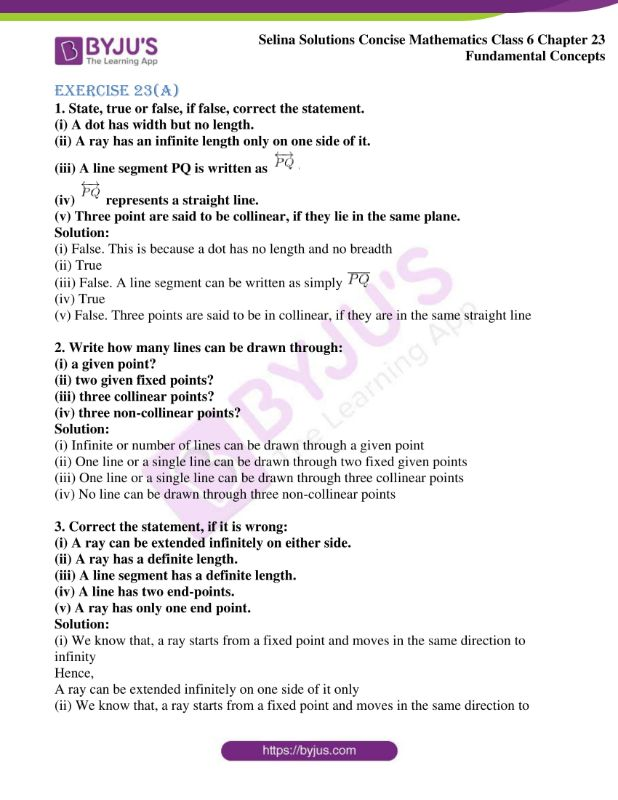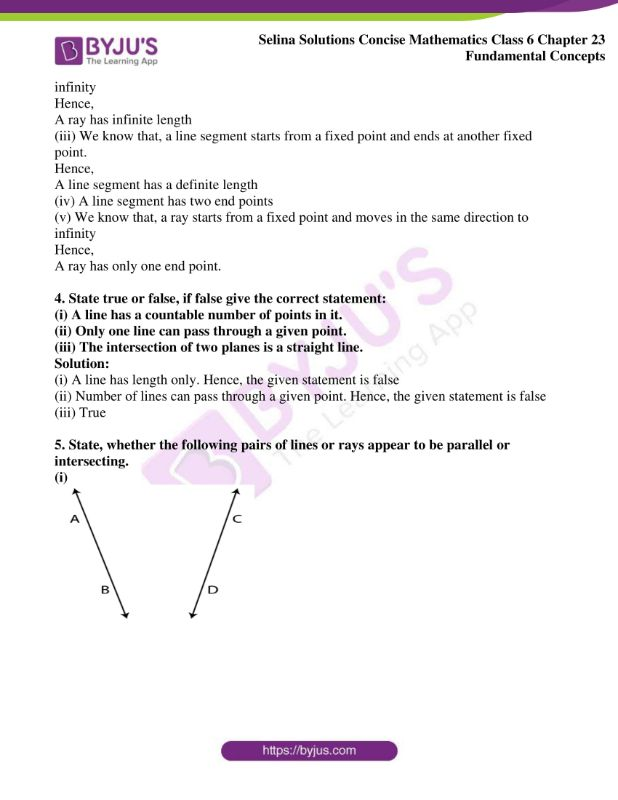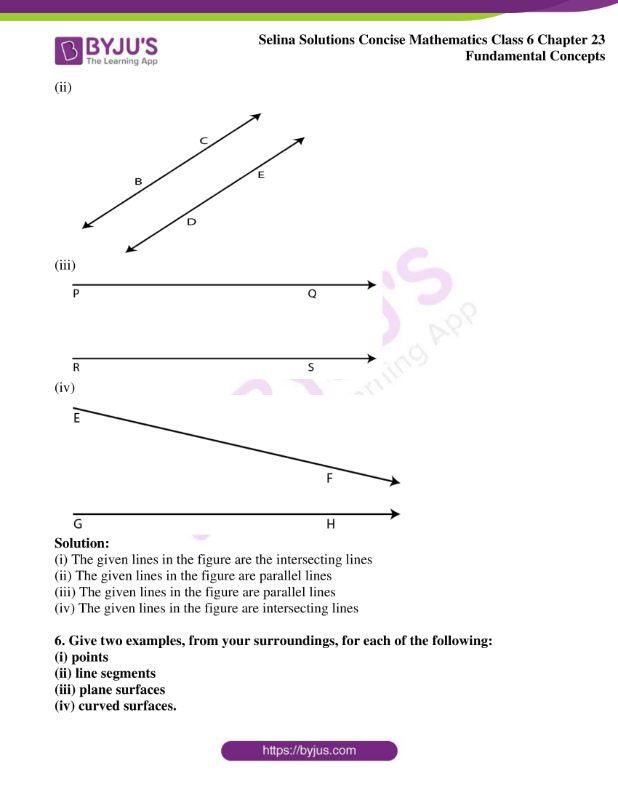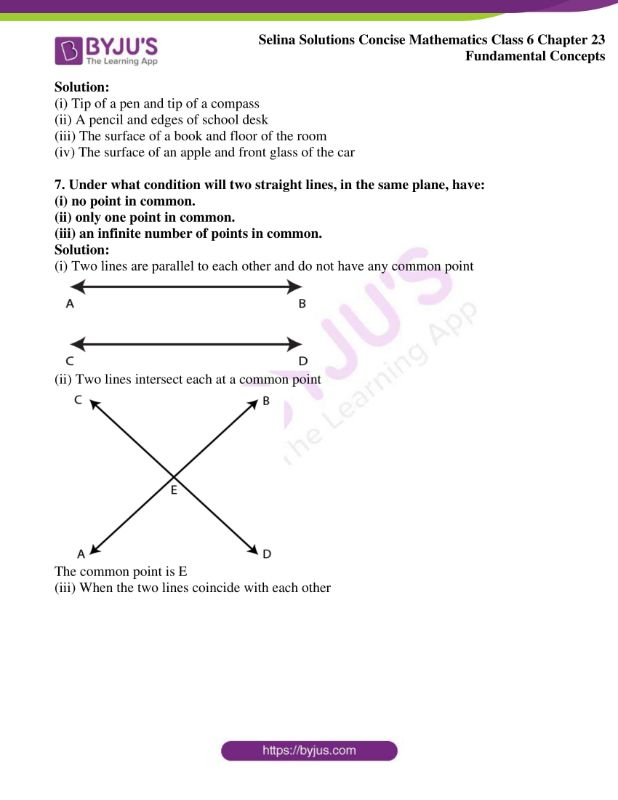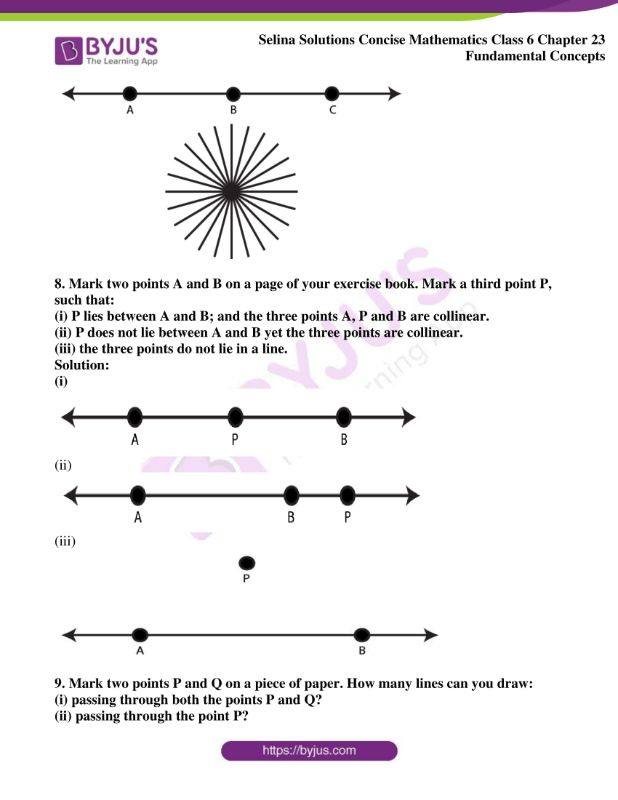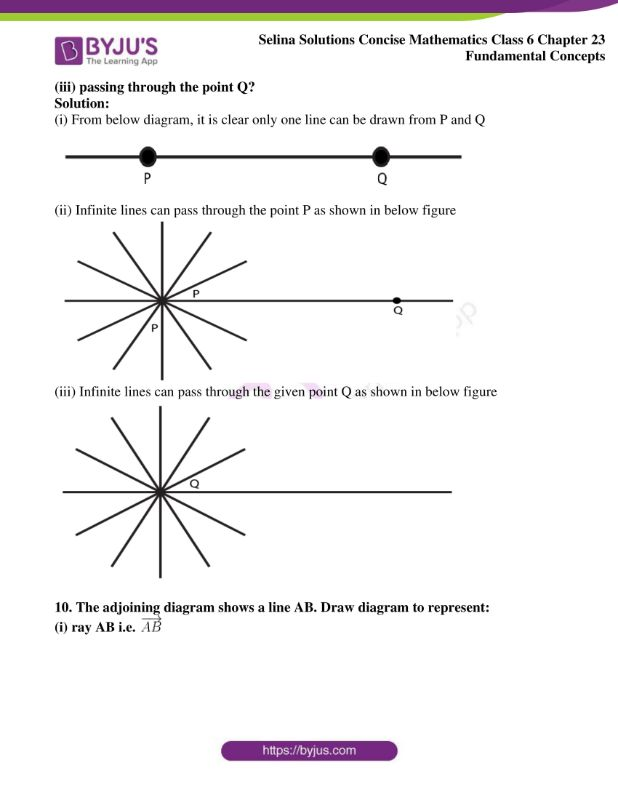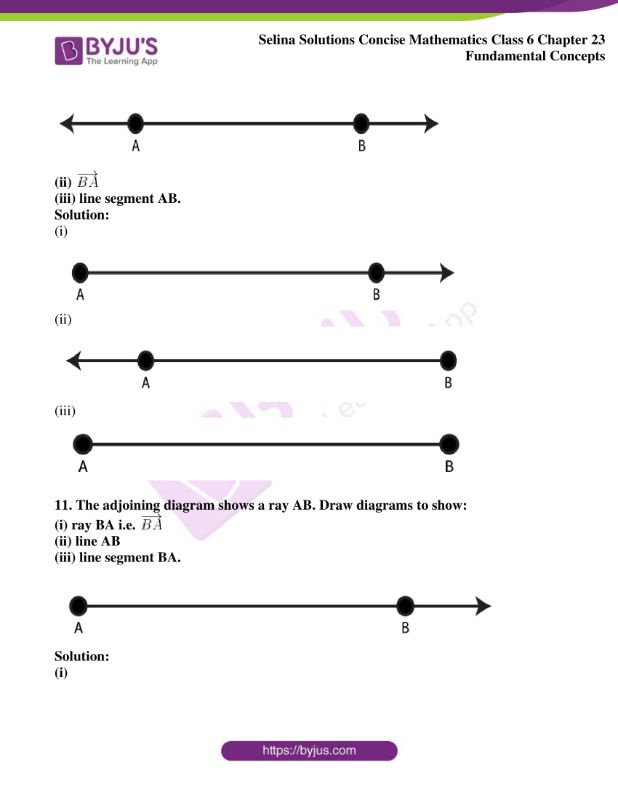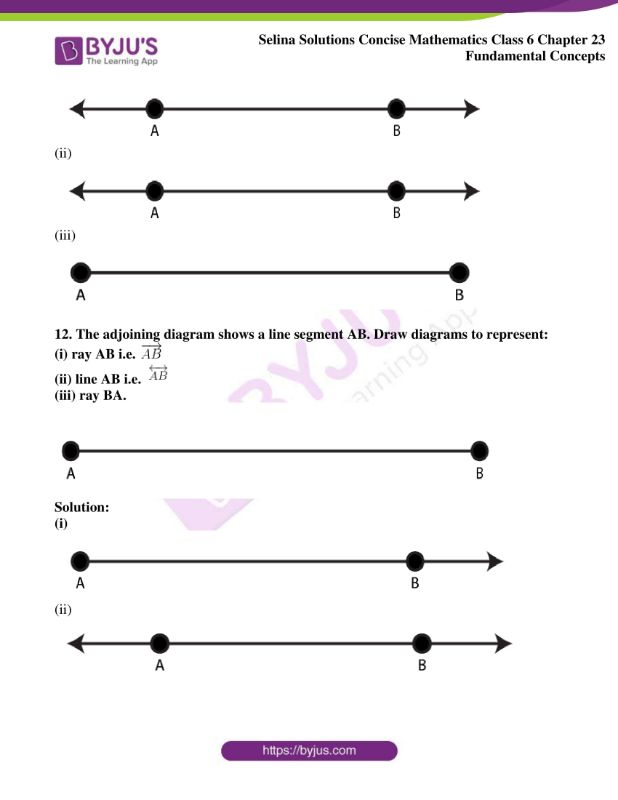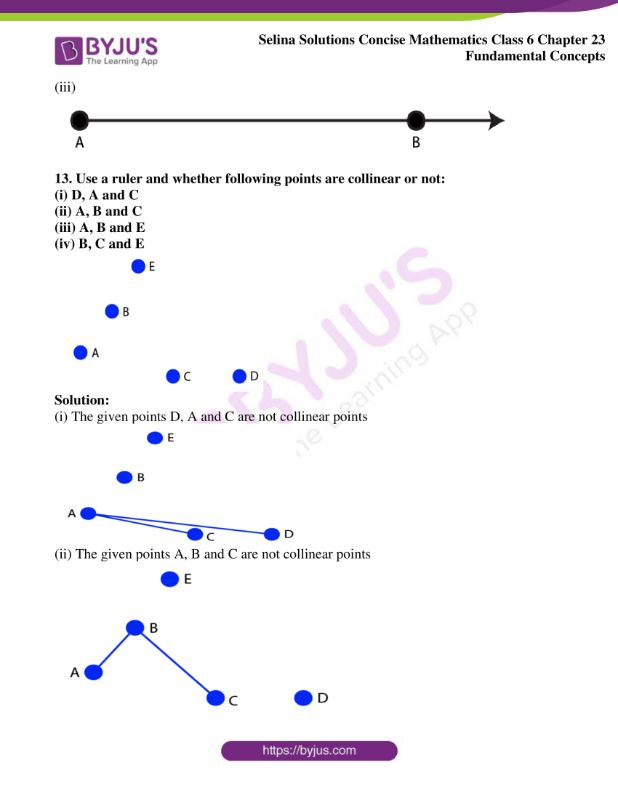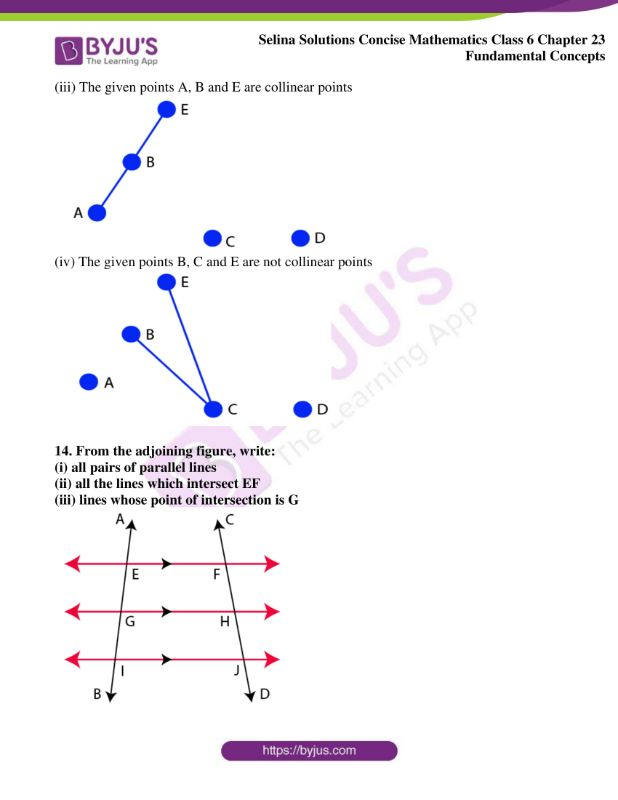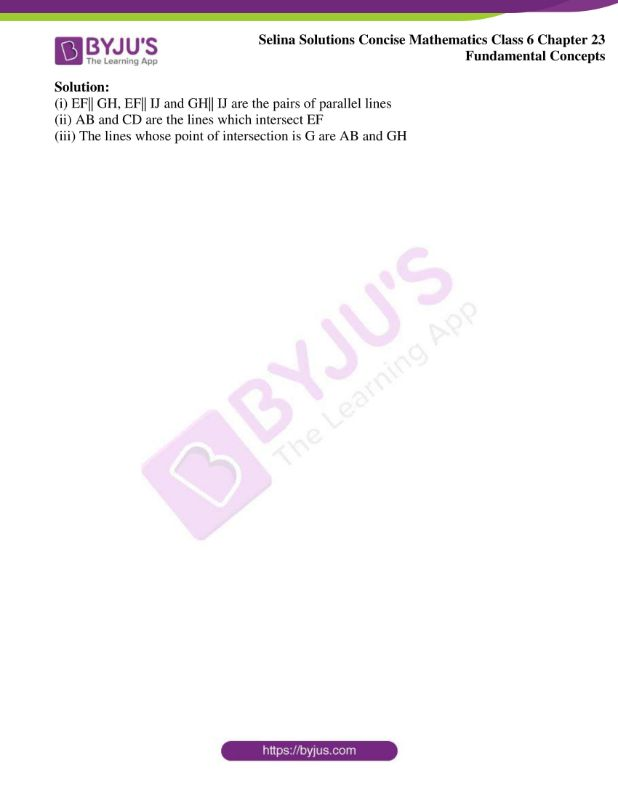### Access Selina Solutions Concise Mathematics Class 6 Chapter 23 Fundamental Concepts Exercise 23(A)

Exercise 23(A)

1. State, true or false, if false, correct the statement.

(i) A dot has width but no length.

(ii) A ray has an infinite length only on one side of it.

(iii) A line segment PQ is written as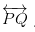(iv)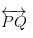represents a straight line.

(v) Three point are said to be collinear, if they lie in the same plane.

Solution:

(i) False. This is because a dot has no length and no breadth

(ii) True

(iii) False. A line segment can be written as simply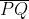(iv) True

(v) False. Three points are said to be in collinear, if they are in the same straight line

2. Write how many lines can be drawn through:

(i) a given point?

(ii) two given fixed points?

(iii) three collinear points?

(iv) three non-collinear points?

Solution:

(i) Infinite or number of lines can be drawn through a given point

(ii) One line or a single line can be drawn through two fixed given points

(iii) One line or a single line can be drawn through three collinear points

(iv) No line can be drawn through three non-collinear points

3. Correct the statement, if it is wrong:

(i) A ray can be extended infinitely on either side.

(ii) A ray has a definite length.

(iii) A line segment has a definite length.

(iv) A line has two end-points.

(v) A ray has only one end point.

Solution:

(i) We know that, a ray starts from a fixed point and moves in the same direction to infinity

Hence,

A ray can be extended infinitely on one side of it only

(ii) We know that, a ray starts from a fixed point and moves in the same direction to infinity

Hence,

A ray has infinite length

(iii) We know that, a line segment starts from a fixed point and ends at another fixed point.

Hence,

A line segment has a definite length

(iv) A line segment has two end points

(v) We know that, a ray starts from a fixed point and moves in the same direction to infinity

Hence,

A ray has only one end point.

4. State true or false, if false give the correct statement:

(i) A line has a countable number of points in it.

(ii) Only one line can pass through a given point.

(iii) The intersection of two planes is a straight line.

Solution:

(i) A line has length only. Hence, the given statement is false

(ii) Number of lines can pass through a given point. Hence, the given statement is false

(iii) True

5. State, whether the following pairs of lines or rays appear to be parallel or intersecting.

(i)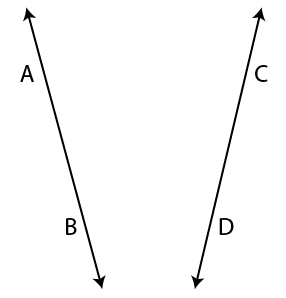(ii)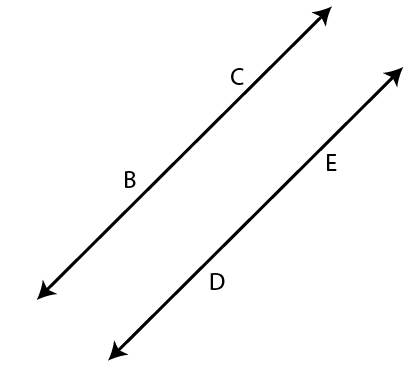(iii)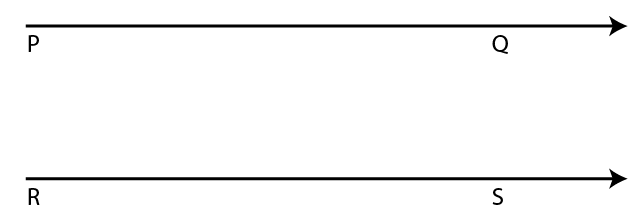(iv)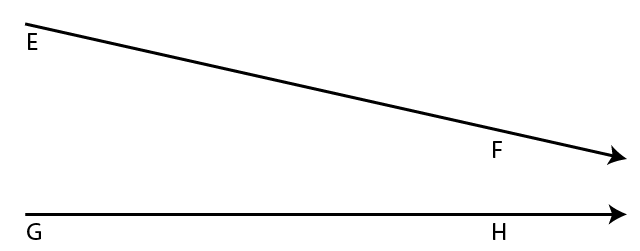Solution:

(i) The given lines in the figure are the intersecting lines

(ii) The given lines in the figure are parallel lines

(iii) The given lines in the figure are parallel lines

(iv) The given lines in the figure are intersecting lines

6. Give two examples, from your surroundings, for each of the following:

(i) points

(ii) line segments

(iii) plane surfaces

(iv) curved surfaces.

Solution:

(i) Tip of a pen and tip of a compass

(ii) A pencil and edges of school desk

(iii) The surface of a book and floor of the room

(iv) The surface of an apple and front glass of the car

7. Under what condition will two straight lines, in the same plane, have:

(i) no point in common.

(ii) only one point in common.

(iii) an infinite number of points in common.

Solution:

(i) Two lines are parallel to each other and do not have any common point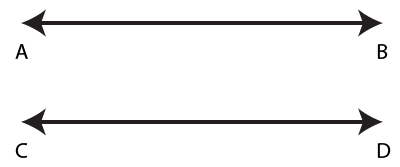(ii) Two lines intersect each at a common point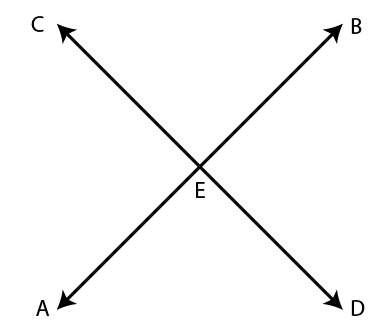The common point is E

(iii) When the two lines coincide with each other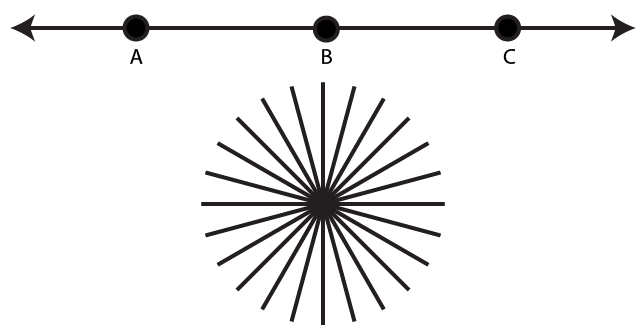8. Mark two points A and B on a page of your exercise book. Mark a third point P, such that:

(i) P lies between A and B; and the three points A, P and B are collinear.

(ii) P does not lie between A and B yet the three points are collinear.

(iii) the three points do not lie in a line.

Solution:

(i)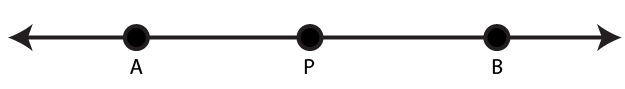(ii)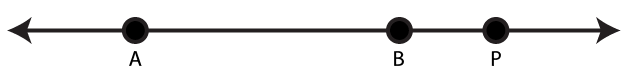(iii)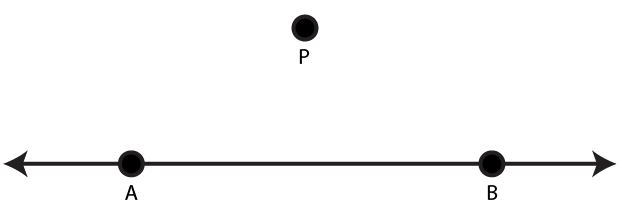9. Mark two points P and Q on a piece of paper. How many lines can you draw:

(i) passing through both the points P and Q?

(ii) passing through the point P?

(iii) passing through the point Q?

Solution:

(i) From below diagram, it is clear only one line can be drawn from P and Q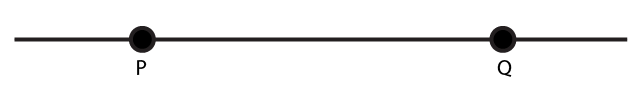(ii) Infinite lines can pass through the point P as shown in below figure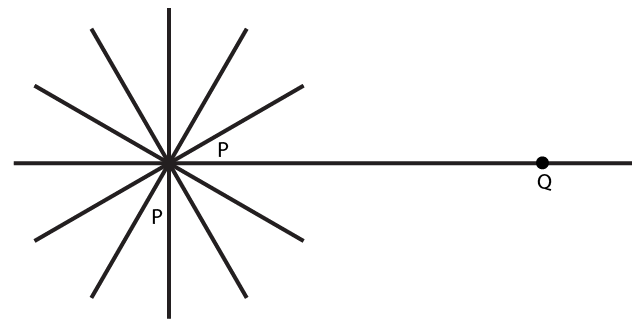(iii) Infinite lines can pass through the given point Q as shown in below figure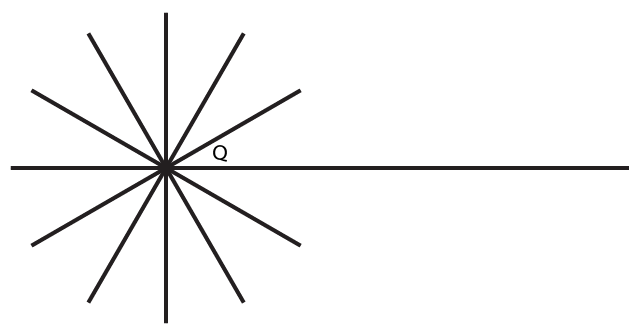10. The adjoining diagram shows a line AB. Draw diagram to represent:

(i) ray AB i.e.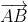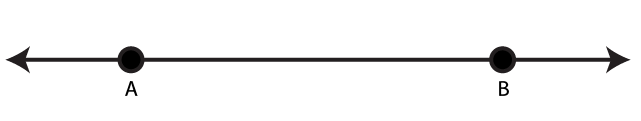(ii)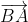(iii) line segment AB.

Solution:

(i)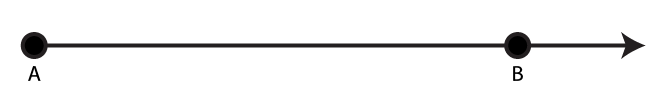(ii)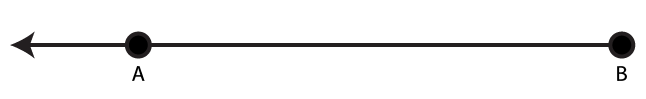(iii)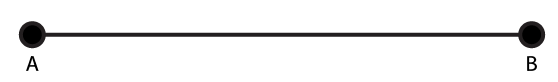11. The adjoining diagram shows a ray AB. Draw diagrams to show:

(i) ray BA i.e.(ii) line AB

(iii) line segment BA.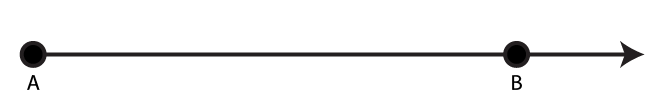Solution:

(i)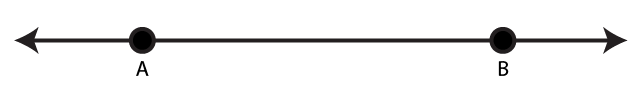(ii)(iii)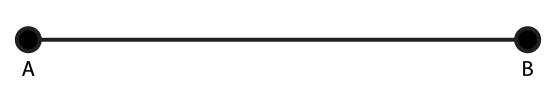12. The adjoining diagram shows a line segment AB. Draw diagrams to represent:

(i) ray AB i.e.(ii) line AB i.e.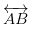(iii) ray BA.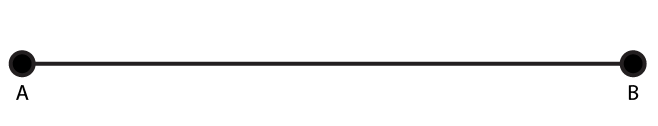Solution:

(i)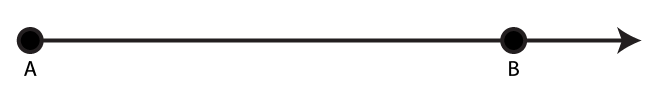(ii)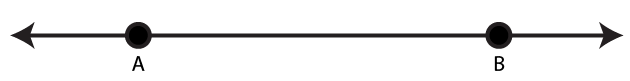(iii)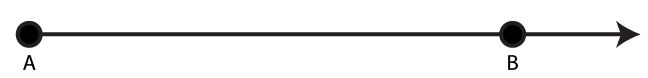13. Use a ruler and whether following points are collinear or not:

(i) D, A and C

(ii) A, B and C

(iii) A, B and E

(iv) B, C and E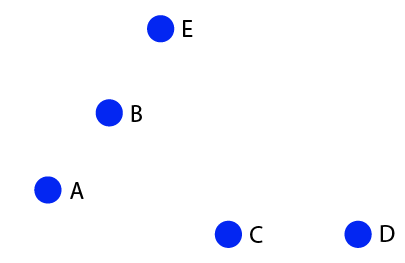Solution:

(i) The given points D, A and C are not collinear points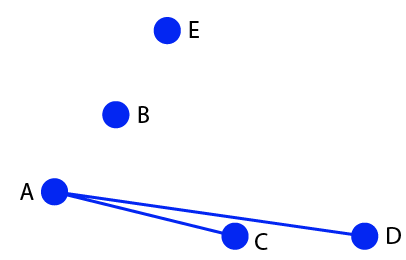(ii) The given points A, B and C are not collinear points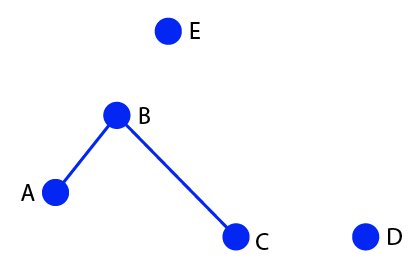(iii) The given points A, B and E are collinear points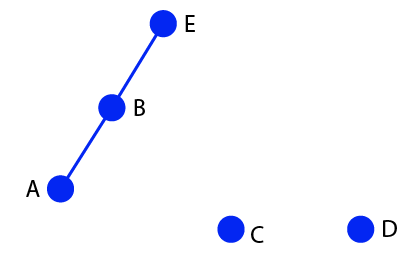(iv) The given points B, C and E are not collinear points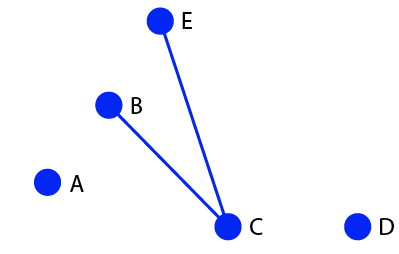14. From the adjoining figure, write:

(i) all pairs of parallel lines

(ii) all the lines which intersect EF

(iii) lines whose point of intersection is G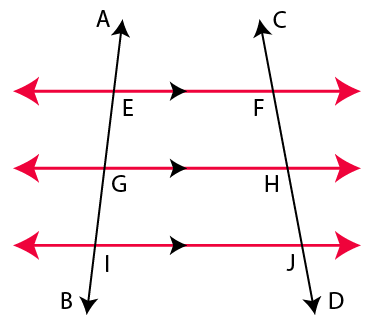Solution:

(i) EF|| GH, EF|| IJ and GH|| IJ are the pairs of parallel lines

(ii) AB and CD are the lines which intersect EF

(iii) The lines whose point of intersection is G are AB and GH